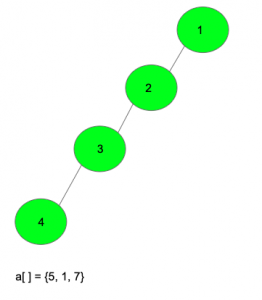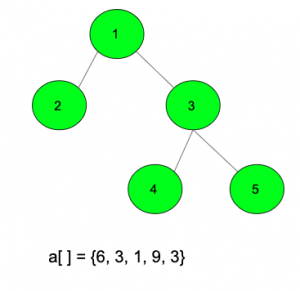# Sum of the distances from every node to all other nodes is maximum

Given a tree with N nodes and N-1 edges with root at 1 and given an array of N-1 integers. The task is to assign weights to the edges in the tree such that the sum of the distances from every node to all other nodes is maximum.

Examples:

Input:Output: 46

Assign the edge 1-2 with weight 5
Assign the edge 2-3 with weight 7
Assign the edge 3-4 with weight 1

The distance of node 1 from the nodes 2, 3, 4 is {5, 5+7, 5+7+1}
The distance of node 2 from the nodes 3, 4 is {7, 7+1}
The distance of node 3 from the node 4 is {1}

Input:Output: 94

## Recommended: Please try your approach on {IDE} first, before moving on to the solution.

Approach: The problem can be solved using Combinations, DFS, DP on trees and Greedy logic. Since we need to assign weights to edges in the tree, hence assigning the maximum weight to the edge which occurs the maximum number of times in all the paths will be the way to get the maximum sum. In order to find the number of times an edge occurs in all the paths possible, we need to know the number of nodes on both the side of the edge. Let c1 and c2 be the count of the number of nodes on the left and right side, then the number of times the edge occurs in all paths will be c1 * c2. Sort all the possible values of c1 * c2 in ascending order. Assign the maximum weight to the maximum c1 * c2 value, and to the others in the same way. We can follow the below steps to get the number of nodes on the left side and on the right side of an edge:

• Run a dfs starting from the root, and initialize a dp[] array which stores the count of the nodes in the subtree of a given node.
• Iterate for every possible edge, and find the number of nodes in the both the side of the edges.
• To find the number of nodes in both the sides, find out the smaller value of dp[node1] or dp[node2], where node1 and node2 are the nodes on the either side of the edge
• If one side has min(dp[node1], dp[node2]), then the other side will have (N – min(dp[node1], dp[node2])).

Below is the implementation of the above approach:

## C++

 `// C++ program to implement the ` `// above approach ` `#include ` `using` `namespace` `std; ` ` `  `// Fucntion to add an edge to the tree ` `void` `addEdge(vector >& edges, ` `             ``list<``int``>* tree, ``int` `x, ``int` `y) ` `{ ` `    ``edges.push_back({ x, y }); ` `    ``tree[x].push_back(y); ` `    ``tree[y].push_back(x); ` `} ` ` `  `// Function to run DFS and calculate the ` `// height of the subtree below it ` `void` `dfs(vector >& edges, list<``int``>* tree, ` `         ``int` `node, ``int` `parent, ``int` `dp[]) ` `{ ` `    ``// Initially initialize with 1 ` `    ``dp[node] = 1; ` ` `  `    ``// Traverse for all nodes connected to node ` `    ``for` `(``auto` `it : tree[node]) { ` `        ``// If node is not parent ` `        ``// then recall dfs ` `        ``if` `(it != parent) { ` `            ``dfs(edges, tree, it, node, dp); ` ` `  `            ``// Add the size of the ` `            ``// subtree beneath it ` `            ``dp[node] += dp[it]; ` `        ``} ` `    ``} ` `} ` ` `  `// Function to assign weights to edges ` `// to maximize the final sum ` `int` `maximizeSum(``int` `a[], vector >& edges, ` `                ``list<``int``>* tree, ``int` `n) ` `{ ` ` `  `    ``// Initialize it which stores the ` `    ``// height of the subtree beneath it ` `    ``int` `dp[n + 1] = { 0 }; ` ` `  `    ``// Call the DFS function to ` `    ``dfs(edges, tree, 1, 0, dp); ` ` `  `    ``// Sort the given array ` `    ``sort(a, a + (n - 1)); ` ` `  `    ``// Stores the number of times an ` `    ``// edge is part of a path ` `    ``vector<``int``> ans; ` ` `  `    ``// Iterate for all edges and find the ` `    ``// number of nodes on the left and on the right ` `    ``for` `(``auto` `it : edges) { ` ` `  `        ``// Node 1 ` `        ``int` `x = it.first; ` ` `  `        ``// Node 2 ` `        ``int` `y = it.second; ` ` `  `        ``// If the number of nodes below is less ` `        ``// then the other will be n - dp[node] ` `        ``if` `(dp[x] < dp[y]) { ` `            ``int` `fi = dp[x]; ` `            ``int` `sec = n - dp[x]; ` `            ``ans.push_back(fi * sec); ` `        ``} ` ` `  `        ``// Second condition ` `        ``else` `{ ` `            ``int` `fi = dp[y]; ` `            ``int` `sec = n - dp[y]; ` `            ``ans.push_back(fi * sec); ` `        ``} ` `    ``} ` ` `  `    ``// Sort the number of times ` `    ``// an edges occurs in the path ` `    ``sort(ans.begin(), ans.end()); ` `    ``int` `res = 0; ` ` `  `    ``// Find the summation of all those ` `    ``// paths and return ` `    ``for` `(``int` `i = 0; i < n - 1; i++) { ` `        ``res += ans[i] * a[i]; ` `    ``} ` ` `  `    ``return` `res; ` `} ` ` `  `// Driver code ` `int` `main() ` `{ ` `    ``int` `n = 5; ` `    ``vector > edges; ` ` `  `    ``list<``int``>* tree = ``new` `list<``int``>[n + 1]; ` ` `  `    ``// Add an edge 1-2 in the tree ` `    ``addEdge(edges, tree, 1, 2); ` ` `  `    ``// Add an edge 2-3 in the tree ` `    ``addEdge(edges, tree, 1, 3); ` ` `  `    ``// Add an edge 3-4 in the tree ` `    ``addEdge(edges, tree, 3, 4); ` ` `  `    ``// Add an edge 3-5 in the tree ` `    ``addEdge(edges, tree, 3, 5); ` ` `  `    ``// Array which gives the edges weight ` `    ``// to be assigned ` `    ``int` `a[] = { 6, 3, 1, 9, 3 }; ` ` `  `    ``cout << maximizeSum(a, edges, tree, n); ` `} `

## Python3

 `# Python3 program to implement the ` `# above approach ` ` `  `edges ``=` `[[] ``for` `i ``in` `range``(``100``)] ` `tree ``=` `[[] ``for` `i ``in` `range``(``100``)] ` ` `  `# Function to add an edge to the tree ` `def` `addEdge(x, y): ` `    ``edges.append([x, y]) ` `    ``tree[x].append(y) ` `    ``tree[y].append(x) ` ` `  `# Function to run DFS and calculate the ` `# height of the subtree below it ` `def` `dfs(node, parent, dp): ` `     `  `    ``# Intially initialize with 1 ` `    ``dp[node] ``=` `1` ` `  `    ``# Traverse for all nodes connected to node ` `    ``for` `it ``in` `tree[node]: ` `         `  `        ``# If node is not parent ` `        ``# then recall dfs ` `        ``if` `(it !``=` `parent): ` `            ``dfs(it, node, dp) ` ` `  `            ``# Add the size of the ` `            ``# subtree beneath it ` `            ``dp[node] ``+``=` `dp[it] ` ` `  `# Function to assign weights to edges ` `# to maximize the final sum ` `def` `maximizeSum(a, n): ` ` `  `    ``# Initialize it which stores the ` `    ``# height of the subtree beneath it ` `    ``dp ``=` `[``0` `for` `i ``in` `range``(n ``+` `1``)] ` ` `  `    ``# Call the DFS function to ` `    ``dfs(``1``, ``0``, dp) ` ` `  `    ``# Sort the given array ` `    ``a ``=` `sorted``(a[:``-``1``]) ` ` `  `    ``# Stores the number of times an ` `    ``# edge is part of a path ` `    ``ans ``=` `[] ` ` `  `    ``# Iterate for all edges and find the ` `    ``# number of nodes on the left and on the right ` `    ``for` `it ``in` `edges: ` ` `  `        ``if` `len``(it) > ``0``: ` ` `  `            ``# Node 1 ` `            ``x ``=` `it[``0``] ` ` `  `            ``# Node 2 ` `            ``y ``=` `it[``1``] ` ` `  `            ``# If the number of nodes below is less ` `            ``# then the other will be n - dp[node] ` `            ``if` `(dp[x] < dp[y]): ` ` `  `                ``fi ``=` `dp[x] ` `                ``sec ``=` `n ``-` `dp[x] ` `                ``ans.append(fi ``*` `sec) ` ` `  `            ``# Second condition ` `            ``else``: ` `                ``fi ``=` `dp[y] ` `                ``sec ``=` `n ``-` `dp[y] ` `                ``ans.append(fi ``*` `sec) ` ` `  `    ``# Sort the number of times ` `    ``# an edges occurs in the path ` `    ``ans ``=` `sorted``(ans) ` `    ``res ``=` `0` ` `  `    ``# Find the summation of all those ` `    ``# paths and return ` `    ``for` `i ``in` `range``(n ``-` `1``): ` `        ``res ``+``=` `ans[i] ``*` `a[i] ` ` `  `    ``return` `res ` ` `  `# Driver code ` `n ``=` `5` ` `  `# Add an edge 1-2 in the tree ` `addEdge(``1``, ``2``) ` ` `  `# Add an edge 2-3 in the tree ` `addEdge(``1``, ``3``) ` ` `  `# Add an edge 3-4 in the tree ` `addEdge(``3``, ``4``) ` ` `  `# Add an edge 3-5 in the tree ` `addEdge(``3``, ``5``) ` ` `  `# Array which gives the edges weight ` `# to be assigned ` `a ``=` `[``6``, ``3``, ``1``, ``9``, ``3``] ` `print``(maximizeSum(a, n)) ` ` `  `# This code is contributed by Mohit Kumar `

Output:

```94
```

Attention reader! Don’t stop learning now. Get hold of all the important DSA concepts with the DSA Self Paced Course at a student-friendly price and become industry ready.

My Personal Notes arrow_drop_upCheck out this Author's contributed articles.

If you like GeeksforGeeks and would like to contribute, you can also write an article using contribute.geeksforgeeks.org or mail your article to contribute@geeksforgeeks.org. See your article appearing on the GeeksforGeeks main page and help other Geeks.

Please Improve this article if you find anything incorrect by clicking on the "Improve Article" button below.

Improved By : mohit kumar 29